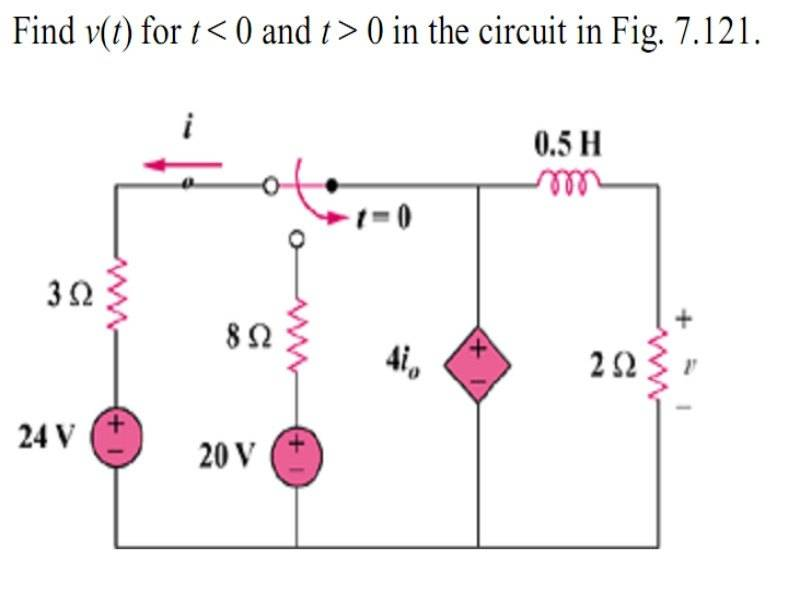# Need help on solving a RL circuit with switch

• Engineering

## Homework Statement## Homework Equations

Find v(t) for t < 0 and t > 0 in the circuit.

## The Attempt at a Solution

I tried to find the i through the inductor for both t=0- and t=infinity, but I did not get the same result as the answer given in the txtbook which is 96e-4t V. According to the answer given from the txtbk, if I'm not wrong, i(t) shud be i(t)=48e-4t A, and then to find v at the 2 ohm resistor, i(t) X 2 and u get v(t).

I do not really understand why, I've been on this qn for 1 hr and stil can't understand it.

Mentor

## Homework Statement## Homework Equations

Find v(t) for t < 0 and t > 0 in the circuit.

## The Attempt at a Solution

I tried to find the i through the inductor for both t=0- and t=infinity, but I did not get the same result as the answer given in the txtbook which is 96e-4t V. According to the answer given from the txtbk, if I'm not wrong, i(t) shud be i(t)=48e-4t A, and then to find v at the 2 ohm resistor, i(t) X 2 and u get v(t).

I do not really understand why, I've been on this qn for 1 hr and stil can't understand it.

What's I_o?

Io is I0, the value should be calculated when t=o-

Mentor
Io is I0, the value should be calculated when t=o-

It's a bit confusing for me, but to clarify... So the switch is opened at t=0? And right before then, there is 1*I_o flowing to the left, and 3*I_o flowing to the right? To add up to the 4*I_o that is supplied by the dependent current source in the middle?

Staff Emeritus